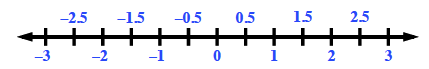### Home > CC1 > Chapter 5 > Lesson 5.3.3 > Problem5-95

5-95.

Draw a number line. Then draw and label a dot to show the position of each of the following numbers.

1.  $2.5$

1.  $\frac{1}{2}$

1.  $−2$

1.  $0.5$

1.  $−1\frac{1}{2}$

1.  $-\frac{3}{4}$

First, look at each number you will need to plot and try to scale the number line.

You may have noticed that most of the numbers are whole or half numbers, so you might like to scale the number line by $0.5\text{ or }\frac{1}{2}.$
You could also scale the number line by $0.25$ or $\frac{1}{4}$.
Be sure that the line includes both positive and negative values!

This is a sample number line. Can you plot the points here?Remember that $0.5$ is equal to $\frac{1}{2}$. Also, remember to label your points!